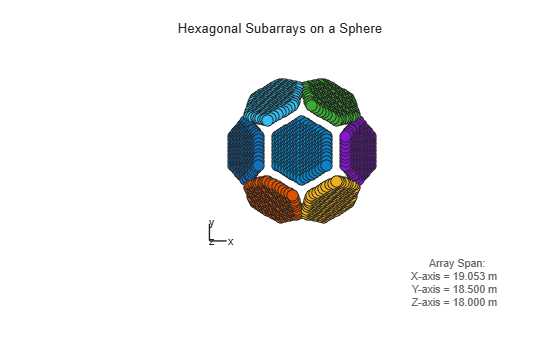# viewArray

System object: phased.ReplicatedSubarray
Package: phased

View array geometry

## Syntax

```viewArray(H) viewArray(H,Name,Value) hPlot = viewArray(___) ```

## Description

`viewArray(H)` plots the geometry of the array specified in `H`.

`viewArray(H,Name,Value)` plots the geometry of the array, with additional options specified by one or more `Name,Value` pair arguments.

`hPlot = viewArray(___)` returns the handles of the array elements in the figure window. All input arguments described for the previous syntaxes also apply here.

## Input Arguments

 `H` Array object.

### Name-Value Arguments

Specify optional comma-separated pairs of `Name,Value` arguments. `Name` is the argument name and `Value` is the corresponding value. `Name` must appear inside quotes. You can specify several name and value pair arguments in any order as `Name1,Value1,...,NameN,ValueN`.

 `Parent` Handle to the axes along which the array geometry is displayed. `ShowNormals` Set this value to `true` to show the normal directions of all elements of the array. Set this value to `false` to plot the elements without showing normal directions. Default: `false` `ShowLocalCoordinates` Logical flag specifying whether to show the local coordinate axes. Default: `true` `ShowAnnotation` Logical flag specifying whether to show the annotations in the UI panel of the figure. Annotation shows aperture size and element spacing based on array axis of array. Default: `true` `Orientation` Orientation of the array, specified as a 3-by-1 column vector containing the rotation angles with respect to the x-, y-, and z-axes of the local coordinate system, respectively. The default value is [0;0;0]. Default: `[0;0;0]` `ShowTaper` Set this value to `true` to specify whether to change the element color brightness in proportion to the element taper magnitude. When this value is set to `false`, all elements are drawn with the same color. Default: `false` `ShowIndex` Vector specifying the element indices to show in the figure. Each number in the vector must be an integer between 1 and the number of elements. You can also specify the value as `'All'` to show indices of all elements of the array or `'None'` to suppress indices. Default: `'None'` `ShowSubarray` Vector specifying the indices of subarrays to highlight in the figure. Each number in the vector must be an integer between 1 and the number of subarrays. You can also specify the value as `'All'` to highlight all subarrays of the array or `'None'` to suppress subarray highlighting. Highlighting uses different colors for different subarrays. Default: `'All'` `Title` Character vector specifying the title of the plot. Default: `'Array Geometry'`

## Output Arguments

 `hPlot` Handles of array elements in figure window.

## Examples

expand all

This example shows how to construct a full array by replicating subarrays.

Create a hexagonal array to use as a subarray.

```Nmin = 9; Nmax = 17; dy = 0.5; dz = 0.5*sin(pi/3); rowlengths = [Nmin:Nmax Nmax-1:-1:Nmin]; numels_hex = sum(rowlengths); stopvals = cumsum(rowlengths); startvals = stopvals-rowlengths+1; pos = zeros(3,numels_hex); rowidx = 0; for m = Nmin-Nmax:Nmax-Nmin rowidx = rowidx+1; idx = startvals(rowidx):stopvals(rowidx); pos(2,idx) = (-(rowlengths(rowidx)-1)/2:... (rowlengths(rowidx)-1)/2) * dy; pos(3,idx) = m*dz; end hexa = phased.ConformalArray('ElementPosition',pos,... 'ElementNormal',zeros(2,numels_hex));```

Arrange copies of the hexagonal array on a sphere.

```radius = 9; az = [-180 -180 -180 -120 -120 -60 -60 0 0 60 60 120 120 180]; el = [-90 -30 30 -30 30 -30 30 -30 30 -30 30 -30 30 90]; numsubarrays = size(az,2); [x,y,z] = sph2cart(deg2rad(az),deg2rad(el),... radius*ones(1,numsubarrays)); ha = phased.ReplicatedSubarray('Subarray',hexa,... 'Layout','Custom',... 'SubarrayPosition',[x; y; z], ... 'SubarrayNormal',[az; el]);```

Display the geometry of the array, highlighting selected subarrays with different colors.

```viewArray(ha,'ShowSubarray',3:2:13,... 'Title','Hexagonal Subarrays on a Sphere'); view(0,90)```